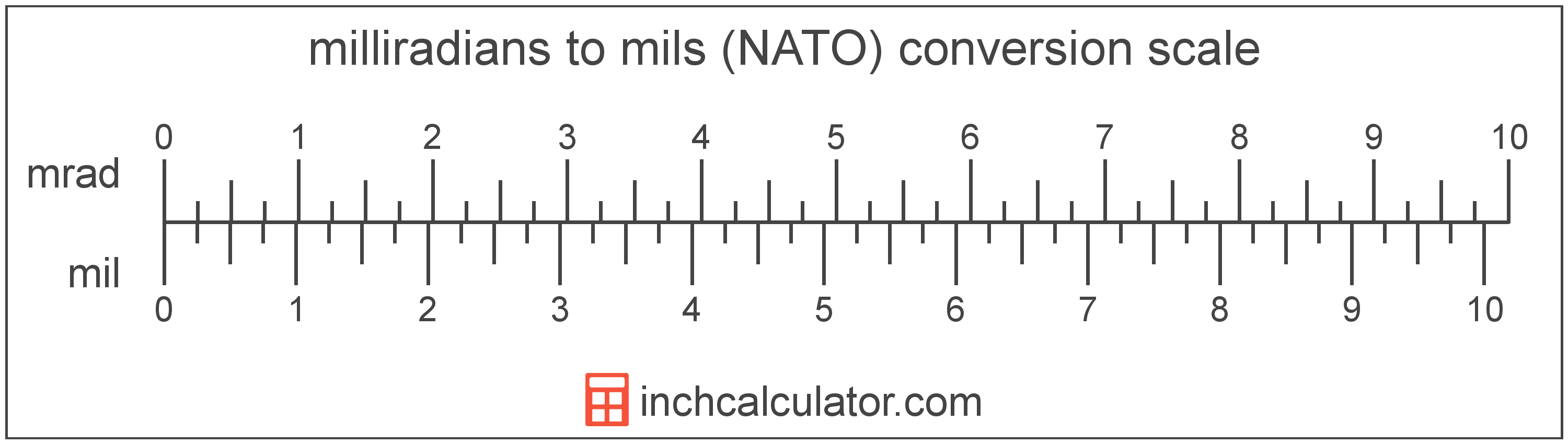# Milliradians to Mils (NATO) Converter

Enter the angle in milliradians below to get the value converted to mils.

Results in Mils:Do you want to convert mils to milliradians?

## How to Convert Milliradians to Mils

To convert a measurement in milliradians to a measurement in mils, multiply the angle by the following conversion ratio: 1.018592 mils/milliradian.

Since one milliradian is equal to 1.018592 mils, you can use this simple formula to convert:

The angle in mils is equal to the angle in milliradians multiplied by 1.018592.

For example, here's how to convert 5 milliradians to mils using the formula above.
mils = (5 mrad × 1.018592) = 5.092958 mil### How Many Mils Are in a Milliradian?

There are 1.018592 mils in a milliradian, which is why we use this value in the formula above.

A milliradian is equal to 1/1,000 of a radian. One milliradian is equal to 0.0573 degrees and there are 6,283 milliradians in a full revolution/circle. The milliradian is also a commonly used unit in mathematics.

The milliradian is a multiple of the radian, which is the SI derived unit for angle. In the metric system, "milli" is the prefix for thousandths, or 10-3. A milliradian is sometimes also referred to as a mil. Milliradians can be abbreviated as mrad; for example, 1 milliradian can be written as 1 mrad.

## What is a Mil?

A mil, short for milliradian, is equal to 1/6,400 of a circle. The mil used by the US military and NATO forces is slightly different than the true value of a milliradian, which is equal to 1/6,283 of a circle.

During World War I the US adopted what is now the NATO mil to replace degrees and minutes for use in artillery sights. They opted to round mils to 6,400 per circle for simplicity at the time. Today, the mil is commonly used to measure adjustment of sights and scopes of firearms.

There is rightfully much confusion as a result of the mil adopted by the US military and NATO being slightly different than the milliradian.

A mil is sometimes also referred to as an angular mil. Mils can be abbreviated as mil; for example, 1 mil can be written as 1 mil.

## Milliradian to Mil Conversion Table

Table showing various milliradian measurements converted to mils.